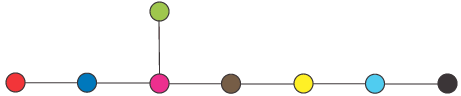# What is E8?

There actually are 4 different but related things called E8.

E8 is first of all the largest exceptional root system, which is a set of vectors in an 8-dimensional real vector space satisfying certain properties. Root systems were classified by Wilhelm Killing in the 1890s. He found 4 infinite classes of Lie algebras, labelled An, Bn, Cn, and Dn, where n=1,2,3.... He also found 5 more exceptional ones: G2, F4, E6, E7, and E8.

The E8 root system consists of all vectors (called roots) (a1,a2,a3,a4,a5,a6,a7,a8) where all ai are integers or all ai are integers plus 1/2, the sum is an even integer, and sum of the squares is 2. An example with all integers is (-1,0,1,0,0,0,0,0) (there are 112 of these) and an example with half-integers is (1/2,1/2,-1/2,-1/2,-1/2,1/2,1/2,-1/2) (there are 128 of these). E8 has 240 roots. The 8 refers to the fact that there are 8 coordinates. See a picture of the E8 root system.

Secondly E8 refers to the root lattice obtained by taking all sums (with integral coefficients) of the vectors in the root system. It consists of all vectors above with all ai integers, or all ai integers plus 1/2, and whose sum is even. The integers of squared length 2 are precisely the roots. This lattice, sometimes called the "8-dimensional diamond lattice", has a number of remarkable properties. It gives most efficient sphere-packing in 8 dimensions, and is also the unique even, unimodular lattice in 8 dimensions. This latter property makes it important in string theory.

Next E8 is a semisimple Lie algebra. A Lie algebra is a vector space, equipped with an operation called the Lie bracket. A simple example is the set of all 2 by 2 matrices. This is a 4-dimensional vector space. The Lie bracket operation is [X,Y]=XY-YX.

E8 is a 248-dimensional Lie algebra. Start with the 8 coordinates above, and add a coordinate for each of the 240 roots of the E8 root system. This vector space has an operation on it, called the Lie bracket: if X,Y are in the Lie algebra so is the Lie bracket [X,Y]. This is like multiplication, except that it is not commutative. Unlike the example of 2 x 2 matrices, it is very hard to write down the formula for the Lie bracket on E8.

This is a complex Lie algebra, i.e. the coordinates are complex numbers. Associated to this Lie algebra is a (complex) Lie group, also called E8. This complex group has (complex) dimension 248. The E8 Lie algebra and group were studied by Elie Cartan in 1894.

Finally E8 is one of three real forms of the the complex Lie group E8. Each of these three real forms has real dimension 248. The group which we are referring to in this web site is the split real form of E8.

### Geometric description of the split real form of E8

Consider 16x16 real matrices X satisfying two conditions. First of all X is a rotation matrix, i.e. its rows and columns are orthonormal. Secondly assume X2=-I. The set of all such matrices V0 is a geometric object (a "real algebraic variety"), and it is 56-dimensional. There is a natural way to add a single circle to this to make a 57-dimensional variety V. (V=Spin(16)/SU(8), and is circle bundle over V0, to anyone keeping score). Finally E8 is a group of symmetries of V.